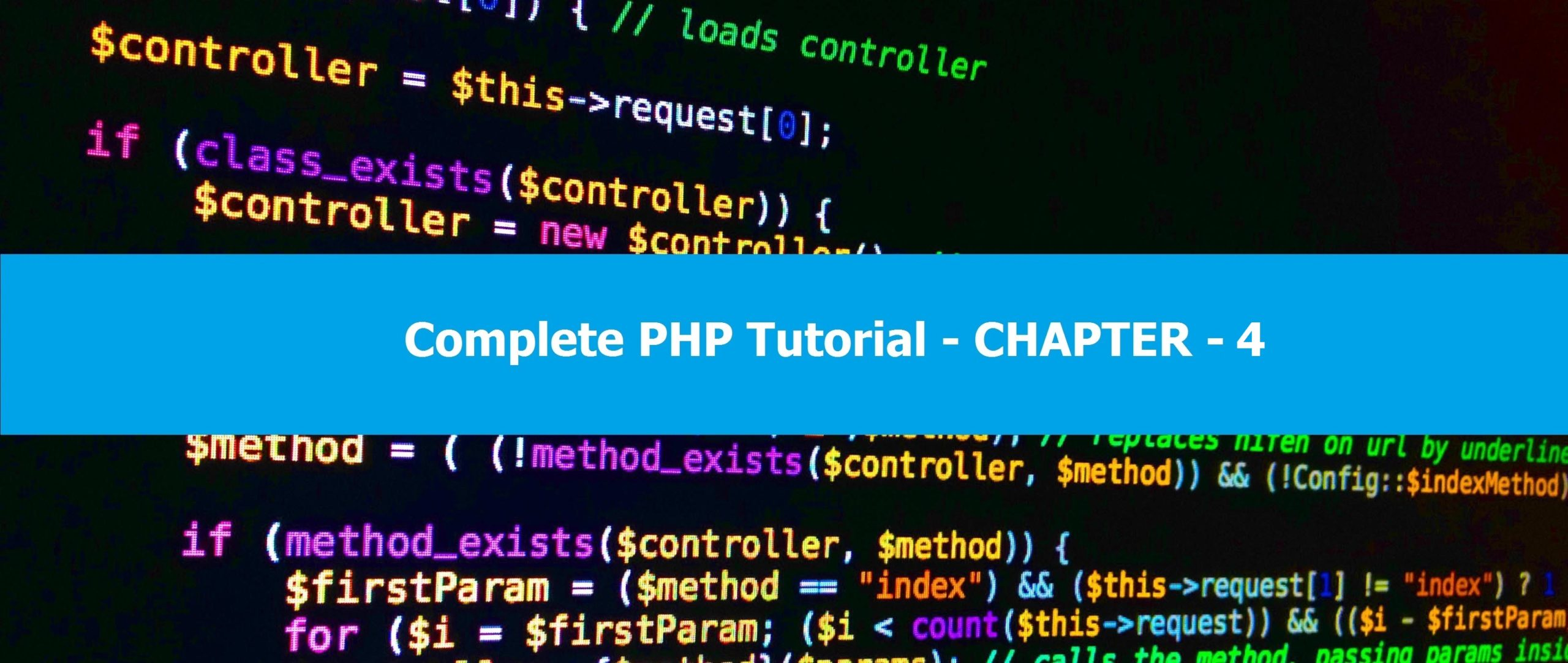# Complete PHP Tutorial – Chapter 4In this chapter, we’ll cover PHP Data types which are of many types as listed below –

### Data Types

A data type is the type of information stored within a variable. PHP has 8 main data types listed here:

• String
• Integer
• Float
• Boolean
• Array
• Object
• NULL
• Resource

Let’s start with an integer. These are whole numbers without decimals that; don’t contain commas or blanks, must not have a decimal point and can be positive or negative.

PHP Code:

<?php
\$inta = 69; //Positive integer
\$intb = -69; //Negative integer
?>

Next is a string where is a “string” of characters. It can be any text within a set of quotation marks (double or single). You can also join two strings together using a dot concatenation operator.

PHP Code:

<?php
\$stringa = “Hello there buddy.”; //You could also replace the ” with ‘.
\$stringb = “Nice weather here.”;

echo \$stringa . \$stringb; //This will add b onto the end of a and output them.
?>

A float (floating point number) is a numeric variable like an integer except it includes a decimal.

PHP Code:

<?php
\$a = 17.6;
?>

A boolean is a variable that can be represented in two possible states: TRUE or FALSE.

PHP Code:

<?php
\$x = TRUE;
\$y = FALSE;
?>

The other variables are a bit advanced for now and will be covered later in the tutorial. It’s good to remember that most variables can be combined with each other and we will go into more detail about this later.

### Variable Scope

The scope of a variable is the part of the script your variable can be referenced or used. There are three types of scope: local, global and static. A variable declared outside of a function has a global scope but can only be used outside of a function.

Variables declared inside of a function with have a local scope and can only be used inside of this function. We will go into parsing local variables into global later using the return function.

PHP Code:

<?php
\$var1 = 500; //This sets a variable with global scope.
function printNum() //Defining a function (will explain this more later)
{
\$var1 = 499; //Sets this variable with a local scope.
echo \$var1; //Will output 499 as it checks for variables with a local scope.
}
printNum(); //Calling the function.
?>

Continue to Next Chapter – Complete PHP Tutorial – Chapter 5

Credit Goes to Mr. RYDIOO